# Bayes' theorem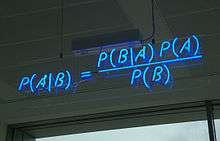A blue neon sign, showing the simple statement of Bayes’ theorem

In probability theory and statistics, Bayes’ theorem (alternatively Bayes’ law or Bayes' rule) describes the probability of an event, based on prior knowledge of conditions that might be related to the event. For example, if cancer is related to age, then, using Bayes’ theorem, a person’s age (prior knowledge) can be used to more accurately assess the probability that they have cancer, compared to the assessment of the probability of cancer made without prior knowledge of the person's age.

One of the many applications of Bayes’ theorem is Bayesian inference, a particular approach to statistical inference. When applied, the probabilities involved in Bayes’ theorem may have different probability interpretations. With the Bayesian probability interpretation the theorem expresses how a subjective degree of belief should rationally change to account for availability of prior related evidence. Bayesian inference is fundamental to Bayesian statistics.

Bayes’ theorem is named after Rev. Thomas Bayes (/ˈbz/; 1701–1761), who first provided an equation that allows new evidence to update beliefs. It was further developed by Pierre-Simon Laplace, who first published the modern formulation in his 1812 “Théorie analytique des probabilités.” Sir Harold Jeffreys put Bayes’ algorithm and Laplace's formulation on an axiomatic basis. Jeffreys wrote that Bayes’ theorem “is to the theory of probability what the Pythagorean theorem is to geometry.”

## Statement of theoremVisualization of Bayes' theorem by the superposition of two decision trees

Bayes' theorem is stated mathematically as the following equation:where A and B are events and P(B) ≠ 0.

• P(A) and P(B) are the probabilities of observing A and B without regard to each other.
• P(A | B), a conditional probability, is the probability of observing event A given that B is true.
• P(B | A) is the probability of observing event B given that A is true.

## Examples

### Cancer at age 65

Suppose that an individual’s probability of having cancer, assigned according to the general prevalence of cancer, is 1%. This is known as the “base rate” or prior (i.e. before being informed about the particular case at hand) probability of having cancer.

Next, suppose that the person is 65 years old. Let us assume that cancer and age are related. The probability that a person has cancer when they are 65 years old is known as the “current probability”, where “current” refers to the theorized situation upon finding out information about the particular case at hand.

Then, suppose that the probability of being 65 years old is 0.2%, and that the probability for a someone diagnosed with cancer to be 65 is 0.5%.

Knowing this, along with the base rate, we can calculate the probability of having cancer as a 65-year-old, which is equal to the probability of being 65 years of age while having cancer, times the probability of having cancer, divided by the probability of being 65 years of age:Possibly more intuitively, in a community of 100,000 people, 1,000 people will have cancer and 200 people will be 65 years old. Of the 1000 people with cancer, only 5 people will be 65 years old. Thus, of the 200 people who are 65 years old, only 5 can be expected to have cancer.

It may come as a surprise that even though being 65 years old increases the risk of having cancer, that person’s probability of having cancer is still fairly low. This is because the base rate of cancer (regardless of age) is low. This illustrates both the importance of base rate, as well as that it is commonly neglected. Base rate neglect leads to serious misinterpretation of statistics; therefore, special care should be taken to avoid such mistakes. Becoming familiar with Bayes’ theorem is one way to combat the natural tendency to neglect base rates.

### Drug testing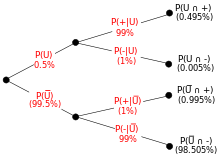Tree diagram illustrating drug testing example. U, Ū, "+" and "−" are the events representing user, non-user, positive result and negative result. Percentages in parentheses are calculated.

Suppose a drug test is 99% sensitive and 99% specific. That is, the test will produce 99% true positive results for drug users and 99% true negative results for non-drug users. Suppose that 0.5% of people are users of the drug. If a randomly selected individual tests positive, what is the probability that he is a user?Despite the apparent accuracy of the test, if an individual tests positive, it is more likely that they do not use the drug than that they do. This again illustrates the importance of base rates, and how the formation of policy can be egregiously misguided if base rates are neglected.

This surprising result arises because the number of non-users is very large compared to the number of users; thus the number of false positives (0.995%) outweighs the number of true positives (0.495%). To use concrete numbers, if 1000 individuals are tested, there are expected to be 995 non-users and 5 users. From the 995 non-users, 0.01 × 995  10 false positives are expected. From the 5 users, 0.99 × 5  5 true positives are expected. Out of 15 positive results, only 5, about 33%, are genuine.

The importance of specificity in this example can be seen by calculating that even if sensitivity is raised to 100% and specificity remains at 99% then the probability of the person being a drug user only rises from 33.2% to 33.4%, but if the sensitivity is held at 99% and the specificity is increased to 99.5% then probability of the person being a drug user rises to about 49.9%.

### A more complicated example

The entire output of a factory is produced on three machines. The three machines account for 20%, 30%, and 50% of the output, respectively. The fraction of defective items produced is this: for the first machine, 5%; for the second machine, 3%; for the third machine, 1%. If an item is chosen at random from the total output and is found to be defective, what is the probability that it was produced by the third machine?

A solution is as follows. Let Ai denote the event that a randomly chosen item was made by the ith machine (for i = 1,2,3). Let B denote the event that a randomly chosen item is defective. Then, we are given the following information:

P(A1) = 0.2,    P(A2) = 0.3,    P(A3) = 0.5.

If the item was made by the first machine, then the probability that it is defective is 0.05; that is, P(B | A1) = 0.05. Overall, we have

P(B | A1) = 0.05,    P(B | A2) = 0.03,    P(B | A3) = 0.01.

To answer the original question, we first find P(B). That can be done in the following way:

P(B) = Σi P(B | Ai) P(Ai) = (0.05)(0.2) + (0.03)(0.3) + (0.01)(0.5) = 0.024.

Hence 2.4% of the total output of the factory is defective.

We are given that B has occurred, and we want to calculate the conditional probability of A3. By Bayes' theorem,

P(A3 | B) = P(B | A3) P(A3)/P(B) = (0.01)(0.50)/(0.024) = 5/24.

Given that the item is defective, the probability that it was made by the third machine is only 5/24. Although machine 3 produces half of the total output, it produces a much smaller fraction of the defective items. Hence the knowledge that the item selected was defective enables us to replace the prior probability P(A3) = 1/2 by the smaller posterior probability P(A3 | B) = 5/24.

Once again, the answer can be reached without recourse to the formula by applying the conditions to any hypothetical number of cases. For example, in 100,000 items produced by the factory, 20,000 will be produced by Machine A, 30,000 by Machine B, and 50,000 by Machine C. Machine A will produce 1000 defective items, Machine B 900, and Machine C 500. Of the total 2400 defective items, only 500, or 5/24 were produced by Machine C.

## InterpretationsA geometric visualisation of Bayes’ theorem. In the table, the values w, x, y and z give the relative weights of each corresponding condition and case. The figures denote the cells of the table involved in each metric, the probability being the fraction of each figure that is shaded. This shows that P (A | B ) P (B ) = P(B | A ) P(A) i.e. P(A | B ) = P(B | A ) P(A )/P (B ) . Similar reasoning can be used to show that P (Ā|B ) = P(B | Ā) P(Ā)/P(B) and so forth.

The interpretation of Bayes’ theorem depends on the interpretation of probability ascribed to the terms. The two main interpretations are described below.

### Bayesian interpretation

In the Bayesian (or epistemological) interpretation, probability measures a “degree of belief.” Bayes’ theorem then links the degree of belief in a proposition before and after accounting for evidence. For example, suppose it is believed with 50% certainty that a coin is twice as likely to land heads than tails. If the coin is flipped a number of times and the outcomes observed, that degree of belief may rise, fall or remain the same depending on the results.

For proposition A and evidence B,

• P (A ), the prior, is the initial degree of belief in A.
• P (A | B ), the “posterior,” is the degree of belief having accounted for B.
• the quotient P(B |A )/P(B) represents the support B provides for A.

For more on the application of Bayes’ theorem under the Bayesian interpretation of probability, see Bayesian inference.

### Frequentist interpretation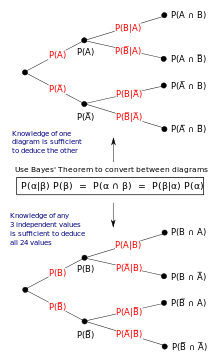Illustration of frequentist interpretation with tree diagrams. Bayest’ theoremm connects conditional probabilities to their inverses.

In the frequentist interpretation, probability measures a “proportion of outcomes.” For example, suppose an experiment is performed many times. P(A) is the proportion of outcomes with property A, and P(B) that with property B. P(B | A ) is the proportion of outcomes with property B out of outcomes with property A, and P(A | B ) the proportion of those with A out of those with B.

The role of Bayes’ theorem is best visualized with tree diagrams, as shown to the right. The two diagrams partition the same outcomes by A and B in opposite orders, to obtain the inverse probabilities. Bayes’ theorem serves as the link between these different partitionings.

#### Example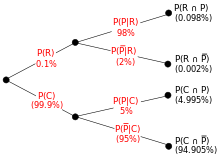Tree diagram illustrating frequentist example. R, C, P and P bar are the events representing rare, common, pattern and no pattern. Percentages in parentheses are calculated. Note that three independent values are given, so it is possible to calculate the inverse tree (see figure above).

An entomologist spots what might be a rare subspecies of beetle, due to the pattern on its back. In the rare subspecies, 98% have the pattern, or P(Pattern | Rare) = 98%. In the common subspecies, 5% have the pattern. The rare subspecies accounts for only 0.1% of the population. How likely is the beetle having the pattern to be rare, or what is P(Rare | Pattern)?

From the extended form of Bayes’ theorem (since any beetle can be only rare or common),## Forms

### Events

#### Simple form

For events A and B, provided that P(B)  0,In many applications, for instance in Bayesian inference, the event B is fixed in the discussion, and we wish to consider the impact of its having been observed on our belief in various possible events A. In such a situation the denominator of the last expression, the probability of the given evidence B, is fixed; what we want to vary is A. Bayes’ theorem then shows that the posterior probabilities are proportional to the numerator:(proportionality over A for given B).

In words: posterior is proportional to prior times likelihood.

If events A1, A2, ..., are mutually exclusive and exhaustive, i.e., one of them is certain to occur but no two can occur together, and we know their probabilities up to proportionality, then we can determine the proportionality constant by using the fact that their probabilities must add up to one. For instance, for a given event A, the event A itself and its complement ¬A are exclusive and exhaustive. Denoting the constant of proportionality by c we haveAdding these two formulas we deduce thator#### Alternative form

Another form of Bayes’ Theorem that is generally encountered when looking at two competing statements or hypotheses is:For an epistemological interpretation:

For proposition A and evidence or background B,

• P(A),the prior probability, is the initial degree of belief in A.
• P(−A), is the corresponding probability of the initial degree of belief against A: 1  P(A) = P(−A)
• P(B | A), the conditional probability or likelihood, is the degree of belief in B, given that the proposition A is true.
• P(B | A), the conditional probability or likelihood, is the degree of belief in B, given that the proposition A is false.
• P(A | B), the posterior probability, is the probability for A after taking into account B for and against A.

#### Extended form

Often, for some partition {Aj} of the sample space, the event space is given or conceptualized in terms of P(Aj) and P(B | Aj). It is then useful to compute P(B) using the law of total probability:In the special case where A is a binary variable:### Random variables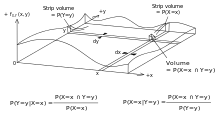Diagram illustrating the meaning of Bayes' theorem as applied to an event space generated by continuous random variables X and Y. Note that there exists an instance of Bayes' theorem for each point in the domain. In practice, these instances might be parametrized by writing the specified probability densities as a function of x and y.

Consider a sample space Ω generated by two random variables X and Y. In principle, Bayes’ theorem applies to the events A = {X = x} and B = {Y = y}. However, terms become 0 at points where either variable has finite probability density. To remain useful, Bayes’ theorem may be formulated in terms of the relevant densities (see Derivation).

#### Simple form

If X is continuous and Y is discrete,If X is discrete and Y is continuous,If both X and Y are continuous,#### Extended form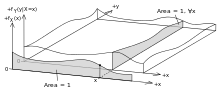Diagram illustrating how an event space generated by continuous random variables X and Y is often conceptualized.

A continuous event space is often conceptualized in terms of the numerator terms. It is then useful to eliminate the denominator using the law of total probability. For fY(y), this becomes an integral:### Bayes’ rule

Main article: Bayes' rule

Bayes' rule is Bayes’ theorem in odds form.whereis called the Bayes factor or likelihood ratio and the odds between two events is simply the ratio of the probabilities of the two events. ThusSo the rule says that the posterior odds are the prior odds times the Bayes factor, or in other words, posterior is proportional to prior times likelihood.

## Derivation

### For events

Bayes’ theorem may be derived from the definition of conditional probability:### For random variables

For two continuous random variables X and Y, Bayes’ theorem may be analogously derived from the definition of conditional density:## History

Bayes’ theorem was named after the Reverend Thomas Bayes (1701–1761), who studied how to compute a distribution for the probability parameter of a binomial distribution (in modern terminology). Bayes’ unpublished manuscript was significantly edited by Richard Price before it was posthumously read at the Royal Society. Price edited Bayes’ major work “An Essay towards solving a Problem in the Doctrine of Chances” (1763), which appeared in “Philosophical Transactions,” and contains Bayes’ Theorem. Price wrote an introduction to the paper which provides some of the philosophical basis of Bayesian statistics. In 1765 he was elected a Fellow of the Royal Society in recognition of his work on the legacy of Bayes.

The French mathematician Pierre-Simon Laplace reproduced and extended Bayes’ results in 1774, apparently quite unaware of Bayes’ work. Stephen Stigler suggested in 1983 that Bayes’ theorem was discovered by Nicholas Saunderson, a blind English mathematician, some time before Bayes; that interpretation, however, has been disputed.

Martyn Hooper and Sharon McGrayne have argued that Richard Price's contribution was substantial:

By modern standards, we should refer to the Bayes–Price rule. Price discovered Bayes’ work, recognized its importance, corrected it, contributed to the article, and found a use for it. The modern convention of employing Bayes’ name alone is unfair but so entrenched that anything else makes little sense.

## Notes

1. Jeffreys, Harold (1973). Scientific Inference (3rd ed.). Cambridge University Press. p. 31. ISBN 978-0-521-18078-8.
2. Stuart, A.; Ord, K. (1994), Kendall's Advanced Theory of Statistics: Volume I—Distribution Theory, Edward Arnold, §8.7.
3. Daniel Kahneman (25 October 2011). Thinking, Fast and Slow. Macmillan. ISBN 978-1-4299-6935-2. Retrieved 8 April 2012.
4. Lee, Peter M. (2012). "Chapter 1". Bayesian Statistics. Wiley. ISBN 978-1-1183-3257-3.
5. "Bayes' Theorem: Introduction". Trinity University.
6. Richard Allen (1999). David Hartley on Human Nature. SUNY Press. pp. 243–4. ISBN 978-0-7914-9451-6. Retrieved 16 June 2013.
7. Bayes, Thomas & Price, Richard (1763). "An Essay towards solving a Problem in the Doctrine of Chance. By the late Rev. Mr. Bayes, communicated by Mr. Price, in a letter to John Canton, A. M. F. R. S." (PDF). Philosophical Transactions of the Royal Society of London. 53 (0): 370–418. doi:10.1098/rstl.1763.0053.
8. Holland, pp. 46–7.
9. Richard Price (1991). Price: Political Writings. Cambridge University Press. p. xxiii. ISBN 978-0-521-40969-8. Retrieved 16 June 2013.
10. Laplace refined Bayes’ theorem over a period of decades:
• Laplace announced his independent discovery of Bayes' theorem in: Laplace (1774) “Mémoire sur la probabilité des causes par les événements,” “Mémoires de l'Académie royale des Sciences de MI (Savants étrangers),” 4: 621–656. Reprinted in: Laplace, “Oeuvres complètes” (Paris, France: Gauthier-Villars et fils, 1841), vol. 8, pp. 27–65. Available on-line at: Gallica. Bayes’ theorem appears on p. 29.
• Laplace presented a refinement of Bayes’ theorem in: Laplace (read: 1783 / published: 1785) “Mémoire sur les approximations des formules qui sont fonctions de très grands nombres,” “Mémoires de l'Académie royale des Sciences de Paris,” 423–467. Reprinted in: Laplace, “Oeuvres complètes” (Paris, France: Gauthier-Villars et fils, 1844), vol. 10, pp. 295–338. Available on-line at: Gallica. Bayes’ theorem is stated on page 301.
• See also: Laplace, “Essai philosophique sur les probabilités” (Paris, France: Mme. Ve. Courcier [Madame veuve (i.e., widow) Courcier], 1814), page 10. English translation: Pierre Simon, Marquis de Laplace with F. W. Truscott and F. L. Emory, trans., “A Philosophical Essay on Probabilities” (New York, New York: John Wiley & Sons, 1902), page 15.
11. Daston, Lorraine (1988). Classical Probability in the Enlightenment. Princeton Univ Press. p. 268. ISBN 0-691-08497-1.
12. Stigler, Stephen M (1983). "Who Discovered Bayes' Theorem?". The American Statistician. 37 (4): 290–296. doi:10.1080/00031305.1983.10483122.
13. De Vaux, Richard; Velleman, Paul; Bock, David (2016). Stats, Data and Models (4 ed.). Pearson. pp. 380–381. ISBN 978-0-321-98649-8.
14. Edwards, A. W. F. (1986). "Is the Reference in Hartley (1749) to Bayesian Inference?". The American Statistician. 40 (2): 109–110. doi:10.1080/00031305.1986.10475370.
15. Hooper, Martyn (2013). "Richard Price, Bayes' theorem, and God". Significance. 10 (1): 36–39. doi:10.1111/j.1740-9713.2013.00638.x.
16. McGrayne, S. B. (2011). The Theory That Would Not Die: How Bayes’ Rule Cracked the Enigma Code, Hunted Down Russian Submarines & Emerged Triumphant from Two Centuries of Controversy. Yale University Press. ISBN 978-0-300-18822-6.

• Bruss, F. Thomas (2013), “250 years of ‘An Essay towards solving a Problem in the Doctrine of Chance. By the late Rev. Mr. Bayes, communicated by Mr. Price, in a letter to John Canton, A. M. F. R. S.’,” DOI 10.1365/s13291-013-0077-z, Jahresbericht der Deutschen Mathematiker-Vereinigung, Springer Verlag, Vol. 115, Issue 3-4 (2013), 129-133.
• Gelman, A, Carlin, JB, Stern, HS, and Rubin, DB (2003), “Bayesian Data Analysis,” Second Edition, CRC Press.
• Grinstead, CM and Snell, JL (1997), “Introduction to Probability (2nd edition),” American Mathematical Society (free pdf available) .
• Hazewinkel, Michiel, ed. (2001), "Bayes formula", Encyclopedia of Mathematics, Springer, ISBN 978-1-55608-010-4
• McGrayne, SB (2011). The Theory That Would Not Die: How Bayes’ Rule Cracked the Enigma Code, Hunted Down Russian Submarines & Emerged Triumphant from Two Centuries of Controversy. Yale University Press. ISBN 978-0-300-18822-6.
• Laplace, P (1774/1986), “Memoir on the Probability of the Causes of Events,” “Statistical Science” 1(3):364–378.
• Lee, Peter M (2012), “Bayesian Statistics: An Introduction,” 4th edition. Wiley. ISBN 978-1-118-33257-3.
• Puga JL, Krzywinski M, Altman N (31 March 2015). "Bayes' theorem". Nature Methods. 12 (4): 2778.
• Rosenthal, Jeffrey S (2005), “Struck by Lightning: The Curious World of Probabilities.” HarperCollins. (Granta, 2008. ISBN 9781862079960).
• Stigler, SM (1986). "Laplace's 1774 Memoir on Inverse Probability". Statistical Science. 1 (3): 359–363. doi:10.1214/ss/1177013620.
• Stone, JV (2013), download chapter 1 of “Bayes’ Rule: A Tutorial Introduction to Bayesian Analysis”, Sebtel Press, England.
• Bayesian Reasoning for Intelligent People An introduction and tutorial to the use of Bayes' theorem in statistics and cognitive science.